Smartick is an advanced online program that teaches kids math and coding in only 15 min. a day

Dec02

# How to Find the Absolute Value of a Number

In today’s post, we are going to learn how to calculate the absolute value of a number with help from some of the exercises that we have in Smartick.

The absolute value of a whole number coincides with its numerical value without having to think about the sign. It is represented with vertical bars around the number, like this: |x|

For example,|2| represents the absolute value of 2.

To calculate it, it is important to have some familiarity with the representation of integers on a number line. So if you would like to review a bit before we continue, I would recommend this previous post explaining how to place numbers on a number line.

It is also necessary to know how to calculate the opposite value of a number. It is a number that has the same value but opposite sign. For example:

• The opposite value of 2 is -2
• The opposite value of -2 is 2

#### Now We Will Look at How to Calculate the Absolute Value of a Number

• When it is positive, the result will be the same number. For example, |5| = 5
• When it is negative the result will be the opposite number. For example, |-3| = 3
• When it is zero,|0| = 0

As you can see, it always takes a value greater than or equal to zero.

Furthermore, it represents the distance from the number 0. This is easy to see on a number line: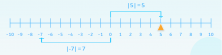Let’s look at some exercises to practice all of this, you’ll see how easy it is!

#### Easy Exercises

The first exercises that we have in Smartick are quite basic. They ask you to calculate the opposite and absolute value, in a written way and then symbolically.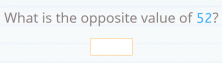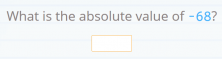#### Comparison Exercises

We also have exercises where you are asked to compare two numbers, one of them being an absolute value.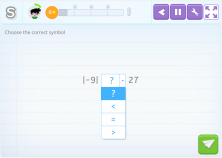Since |-9| = 9 and 9 < 27, then |-9| < 27

So we’ll have to select the “<“ option. Isn’t that easy?

#### More Difficult Comparison Exercises

Finally, we are going to look at some exercises where you are asked to compare two numbers, both of which are absolute values.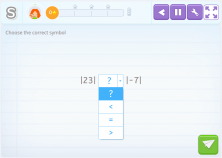Since |23| = 23, |-7| = 7 and 23 > 7, then |23| > |-7|

So we’ll have to select the “>” option. It is a bit more difficult but still easy, right?

Did you like these exercises? Don’t forget to let us know in the comments!